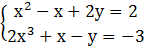### Sample Problem

Is (-1, 0) a solution to?

#### Solution

Substitute -1 for x and 0 for y in each equation.

The left side of the first equation is equal to (-1)2-(-1)+2(0)=1+1=2 which is equal to the right side. So (-1, 0) is a solution of the first equation.

The left side of the second equation is equal to 2(-1)3+(-1)-(0)=-2-1=-3

which is equal to the right side. So (-1, 0) is a solution of the second equation.

Since (-1, 0) fulfill both equations, it is a solution of the system.